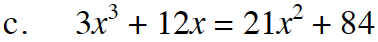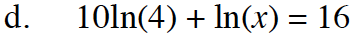### Home > INT3 > Chapter 12 > Lesson 12.2.1 > Problem12-88

12-88.
1. Solve the equations below. Homework Help ✎

1.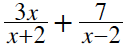= 3

2. (x + 2)2 + (x – 2)2 = (2x)2

3. 3x3 + 12x = 21x2 + 84

4. 10ln(4) + ln(x) = 16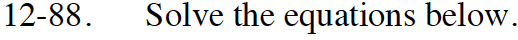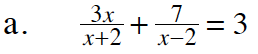Multiply both sides by (x + 2)(x − 2).

3x(x − 2) + 7(x + 2) = 3(x + 2)(x − 2)
3x² − 6x + 7x + 14 = 3(x2 − 4)
3x2 + x + 14 = 3x2 − 12

x = −26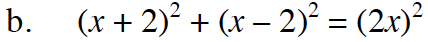x = 10, x = 3